• 我发现智能无人机课程里面讲了无人机建模这方面的理论知识
我发现他PPT里面的图就是全权的《多旋翼飞行器设计与控制实践》这本书上的图，这本书也本身是讲建模仿真的。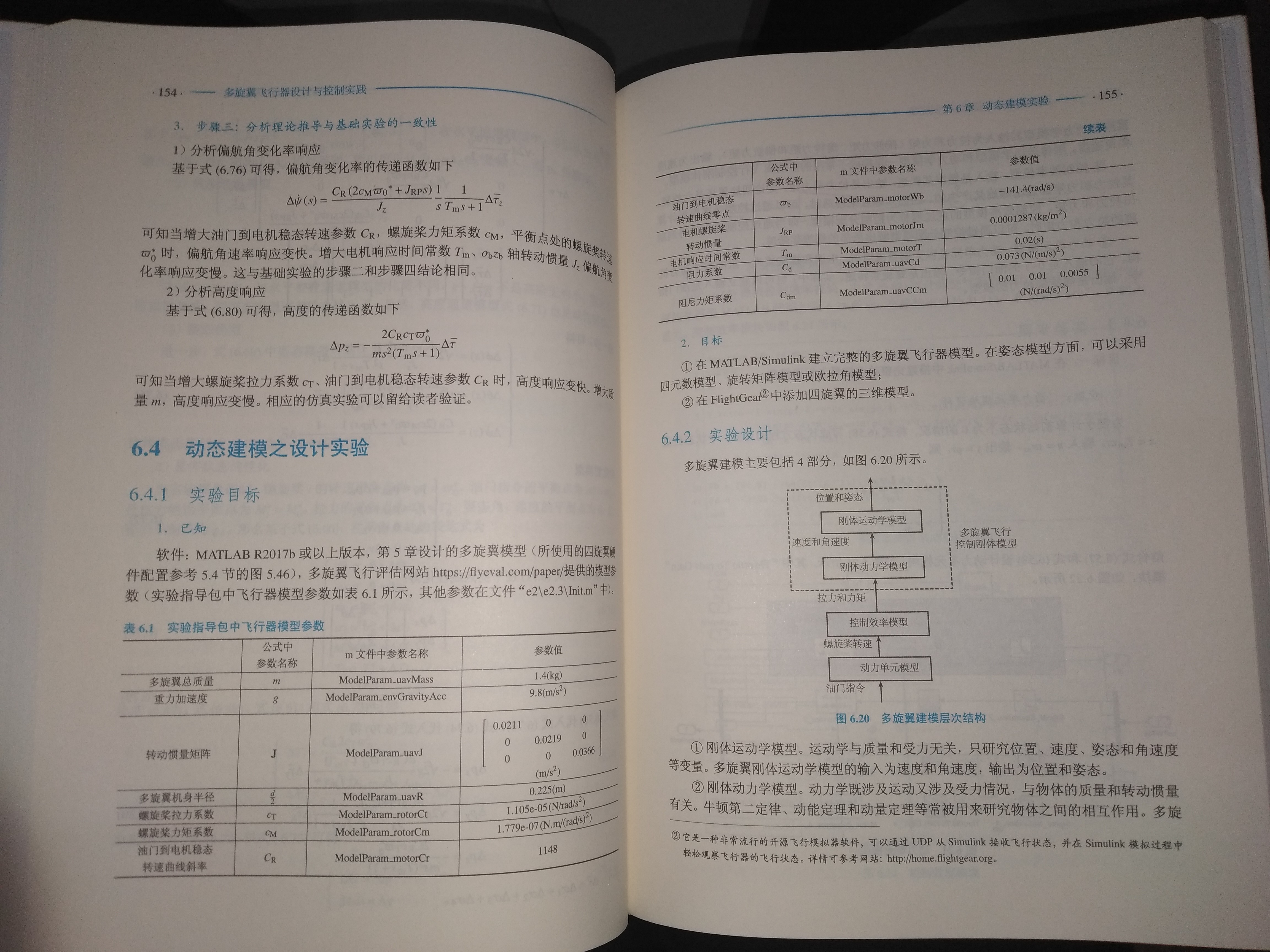========================================

我发现智能无人机课程里面讲了无人机建模这方面的理论知识

只是我之前一直没重视这方面，一直只是在工程上玩玩，别人才是真正专业。

这让我对无人机的理解和把握上升到一个新的境界。真正从理论数学模型的层面去把握，虽然理论之前已经有理解，控制理论，但是并没有建立精确模型。要是真正能建立动力学模型，这个再研究起无人机应该是一个质变，从原先的定量分析可以转变为定量分析，算法验证也应该会方便得多。

我觉得gazebo的无人机仿真  XTDrone的无人机仿真的前提肯定是建立起了精确的无人机动力学模型的，这样才可能去仿真。去进行你的算法验证，我估计无人机的模型的建立现在应该也非常成熟了。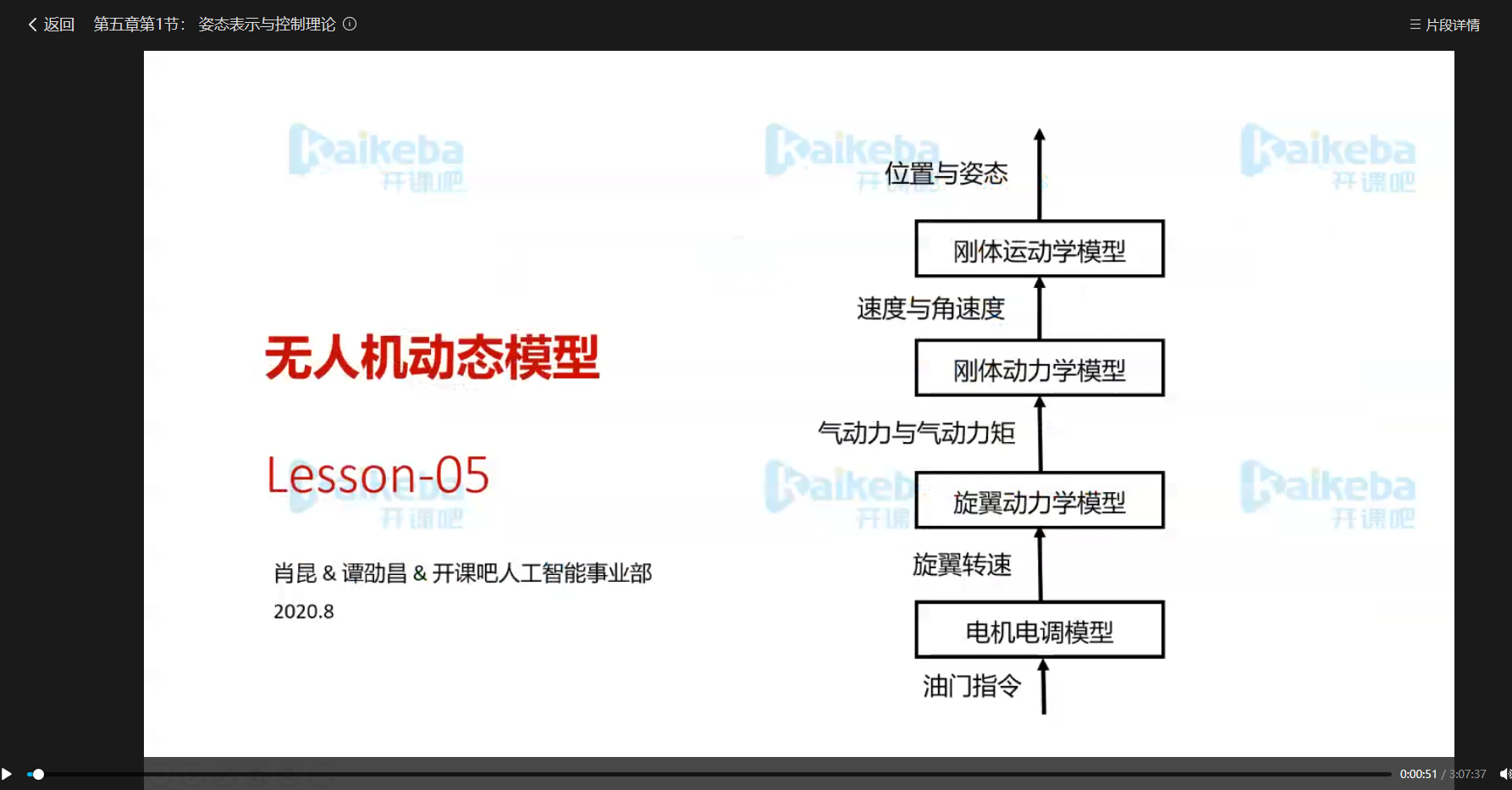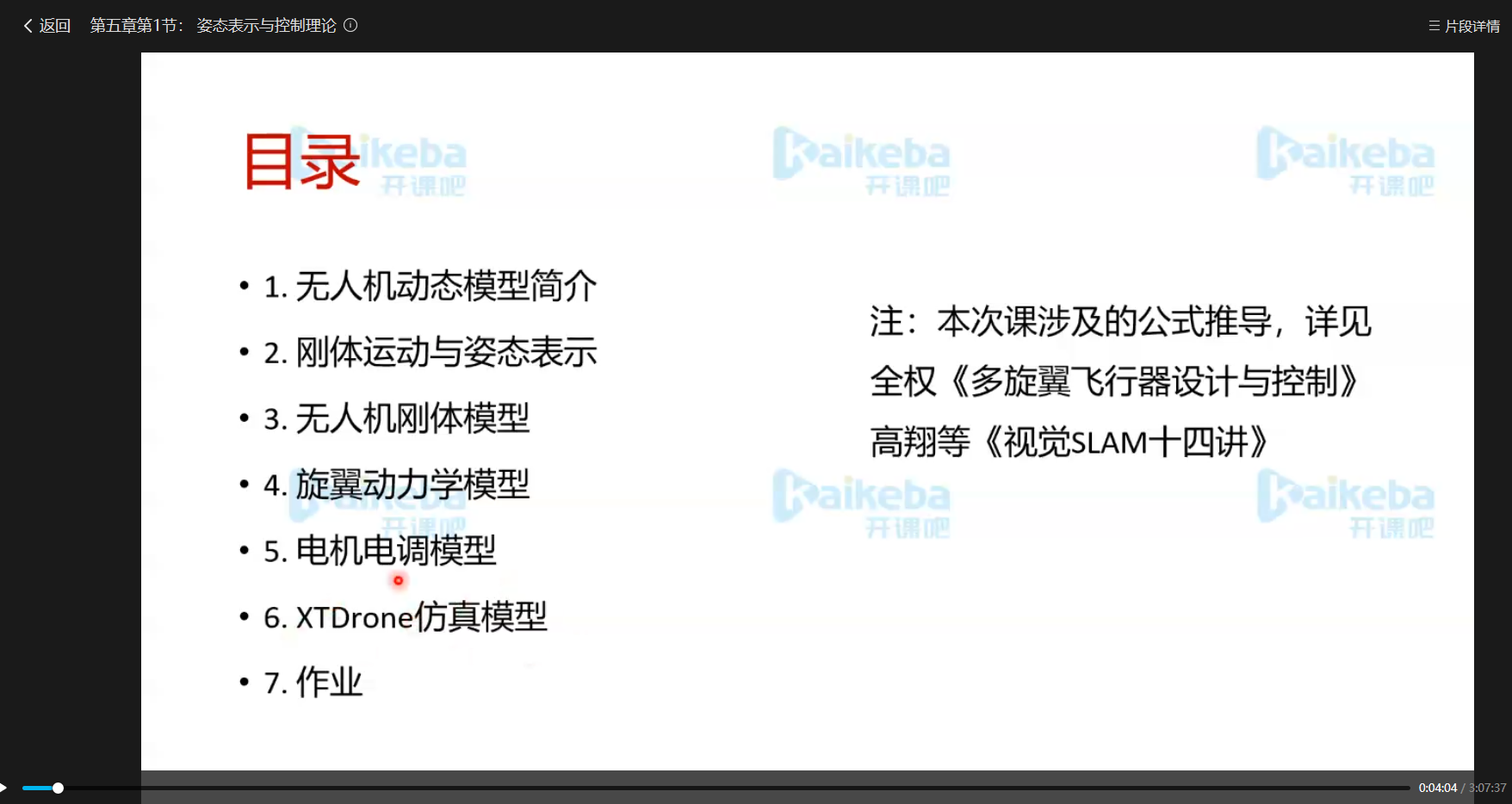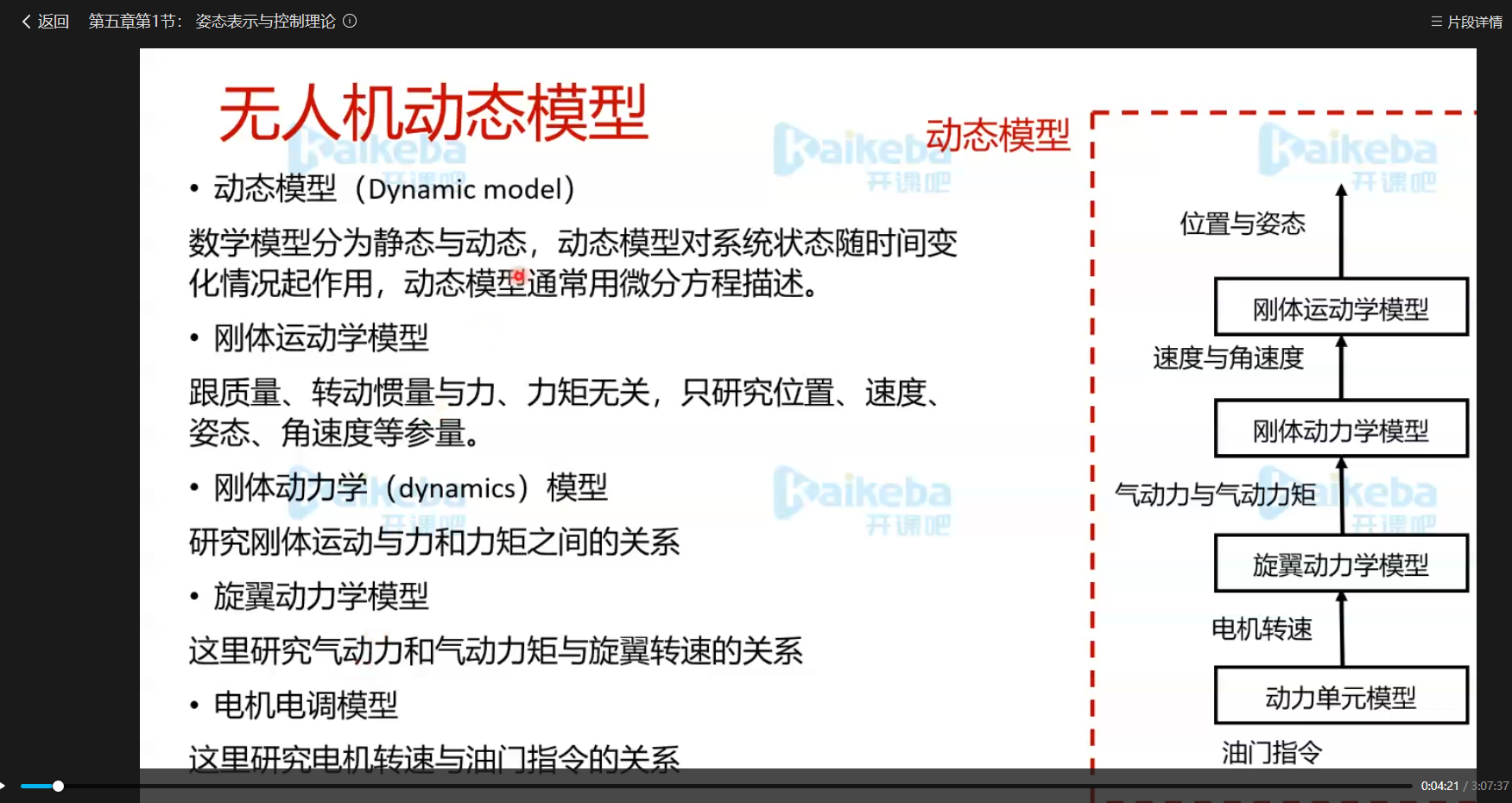展开全文• 多旋翼无人机建模 建模之前我们先分析一下多旋翼无人机有哪些状态量：用于表示线运动的三轴位置、速度和加速度；用于表示角运动的三轴姿态角和姿态角速度；这一共是15个状态量。 首先来看线运动方程的建立过程：...
多旋翼无人机建模
建模之前我们先分析一下多旋翼无人机有哪些状态量：用于表示线运动的三轴位置、速度和加速度；用于表示角运动的三轴姿态角和姿态角速度；这一共是15个状态量。
首先来看线运动方程的建立过程：我们取地理坐标系为北东地(NED)，机体系为前右下：
由牛顿第二定律有：
$F=m a$
即
$F=m \left[ \begin{array}{l}{a_{x}} \\ {a_{y}} \\ {a_{z}}\end{array}\right]$
注意此处的$F$和加速度$a$均是在地理系下的表示($F$表示四个旋翼产生的总升力)，进一步将上式展开可得
$\left[ \begin{array}{c}{0} \\ {0} \\ {m g}\end{array}\right] - \boldsymbol{R}_{b}^{e} \left[ \begin{array}{c}{0} \\ {0} \\ {f_{1}+f_{2}+f_{3}+f_{4}}\end{array}\right]=m \left[ \begin{array}{l}{a_{x}} \\ {a_{y}} \\ {a_{z}}\end{array}\right]$
其中$\boldsymbol{R}_{b}^{e}$表示由机体坐标系到地理坐标系的转换矩阵，由于$f_i,i=1…4$分别表示四个旋翼沿机体$Z$轴负方向产生的升力，故需要将其转换到地理系中；
$\boldsymbol{R}_{b}^{e}=\left[ \begin{array}{ccc}{c \theta c \psi} & {s \phi s \theta c \psi-c \phi s \psi} & {c \phi s \theta c \psi+s \phi s \psi} \\ {c \theta s \psi} & {s \phi s \theta s \psi+c \phi c \psi} & {c \phi s \theta s \psi-s \phi c \psi} \\ {-s \theta} & {s \phi c \theta} & {c \phi c \theta}\end{array}\right]$
联立上式进一步化简即可得到线运动的模型表示：
$\left\{\begin{array}{c}{\ddot{x}=(\mathrm{c} \phi \mathrm{s} \theta \mathrm{c} \psi+\mathrm{s} \phi \mathrm{s} \psi) \frac{-F}{m}} \\ {\ddot{y}=(\mathrm{c} \phi \mathrm{s} \theta \mathrm{s} \psi-\mathrm{s} \phi \mathrm{c} \psi) \frac{-F}{m}} \\ {\ddot{z}=g-(\mathrm{c} \phi \mathrm{c} \theta) \frac{F}{m}}\end{array}\right.$
线运动的建模过程很简单，但是多旋翼不光能移动还能旋转，这个旋转过程就需要刚体的旋转相关知识。
物体如何旋转跟旋翼产生的力矩有关，力矩与力F和距离l有如下公式：$M=F * l$
而这里的力矩又与多旋翼动力布局有关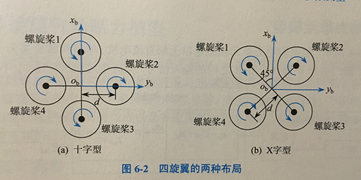如果是十字型的布局：
$F=\sum_{i=1}^{4} T_{i}=c_{\mathrm{T}}\left(w_{1}^{2}+w_{2}^{2}+w_{3}^{2}+w_{4}^{2}\right)$
$\left\{\begin{array}{l}{\tau_{x}=d c_{\mathrm{T}}\left(-w_{2}^{2}+w_{4}^{2}\right)} \\ {\tau_{y}=d c_{\mathrm{T}}\left(w_{1}^{2}-w_{3}^{2}\right)} \\ {\tau_{z}=c_{\mathrm{M}}\left(w_{1}^{2}-w_{2}^{2}+w_{3}^{2}-w_{4}^{2}\right)}\end{array}\right.$
写成矩阵形式如下：
$\left[ \begin{array}{l}{F} \\ {\tau_{x}} \\ {\tau_{y}} \\ {\tau_{z}}\end{array}\right]=\left[ \begin{array}{cccc}{c_{\mathrm{T}}} & {c_{\mathrm{T}}} & {c_{\mathrm{T}}} & {c_{\mathrm{T}}} \\ {0} & {-d c_{\mathrm{T}}} & {0} & {d c_{\mathrm{T}}} \\ {d c_{\mathrm{T}}} & {0} & {-d c_{\mathrm{T}}} & {0} \\ {c_{\mathrm{M}}} & {-c_{\mathrm{M}}} & {c_{\mathrm{M}}} & {-c_{\mathrm{M}}}\end{array}\right] \left[ \begin{array}{c}{w_{1}^{2}} \\ {w_{2}^{2}} \\ {w_{3}^{2}} \\ {w_{4}^{2}}\end{array}\right]$
对X型动力布局：
$F=\sum_{i=1}^{4} T_{i}=c_{\mathrm{T}}\left(w_{1}^{2}+\varpi_{2}^{2}+\varpi_{3}^{2}+\varpi_{4}^{2}\right)$
$\left\{\begin{array}{c}{\tau_{x}=d c_{\mathrm{T}}\left(\sqrt{2} \omega_{1}^{2} / 2-\sqrt{2} \omega_{2}^{2} / 2-\sqrt{2} \omega_{3}^{2} / 2+\sqrt{2} \omega_{4}^{2} / 2\right)} \\ {\tau _{y}=d c_{\mathrm{T}}\left(\sqrt{2} \omega_{1}^{2} / 2+\sqrt{2} \omega_{2}^{2} / 2-\sqrt{2} \omega_{3}^{2} / 2-\sqrt{2} \omega_{4}^{2} / 2\right)} \\ {\tau _{z}=c_{\mathrm{M}}\left(\omega_{1}^{2}-\omega_{2}^{2}+\omega_{3}^{2}-\omega_{4}^{2}\right)}\end{array}\right.$
上式中$F$为四个旋翼产生的总升力，$\tau _x ,\tau _y ,\tau _z$分别表示旋翼产生的作用在三个姿态通道的控制力矩。
根据欧拉方程$M=J \varepsilon+\omega \times J \omega$
其中$\varepsilon$表示角加速度，$\omega$表示角速度，$M$表示姿态通道控制力矩；
欧拉角速度
$\dot{\boldsymbol{\Theta}}=\left[ \begin{array}{c}{\dot{\phi}} \\ {\dot{\theta}} \\ {\dot{\psi}}\end{array}\right]$
与机体角速度
${\boldsymbol{\Omega}}=\left[ \begin{array}{c}{w_x} \\ {w_y} \\ {w_z}\end{array}\right]$
之间的关系为
$\dot{\boldsymbol{\Theta}}=\boldsymbol{W} \boldsymbol{\Omega}$
$W=\left[ \begin{array}{ccc}{1} & {\tan \theta \sin \phi} & {\tan \theta \cos \phi} \\ {0} & {\cos \phi} & {-\sin \phi} \\ {0} & {\sin \phi / \cos \theta} & {\cos \phi / \cos \theta}\end{array}\right]$假设多旋翼无人机姿态角在小角度内变化，则可认为$W=I$，即$\dot{\boldsymbol{\Theta}}= \boldsymbol{\Omega}$
角加速度为$\left[ \begin{array}{c}{\ddot{\phi}} \\ {\ddot{\theta}} \\ {\ddot{\psi}}\end{array}\right]$
转动惯量矩阵为
$\mathbf{J}=\left[ \begin{array}{ccc}{J_{x x}} & {-J_{x y}} & {-J_{x z}} \\ {-J_{y x}} & {J_{y y}} & {-J_{y z}} \\ {-J_{z x}} & {-J_{z y}} & {J_{z z}}\end{array}\right]$
其中$J_{xy}=J_{yx}，J_{xz}=J_{zx}，J_{y z}=J_{z y}$,对于标准多旋翼这样的中心对成物体，有$J_{xy}=J_{xz}=J_{y z}=0$,则有
$\left[ \begin{array}{lll}{J_{x x}} & {0} & {0} \\ {0} & {J_{y y}} & {0} \\ {0} & {0} & {J_{z z}}\end{array}\right]$
整理可得
$\left\{\begin{array}{l}{\ddot{\phi}=\dot{\theta} \dot{\psi} \frac{J_{y}-J_{z}}{J_{x}}+\frac{\tau_{x}}{J_{x}}} \\ {\ddot{\theta}=\dot{\phi} \dot{\psi} \frac{J_{z}-J_{x}}{J_{y}}+\frac{\tau_{y}}{J_{y}}} \\ {\ddot{\psi}=\dot{\phi} \dot{\theta} \frac{J_{x}-J_{y}}{J_{z}}+\frac{\tau_{z}}{J_{z}}}\end{array}\right.$
至此，多旋翼的模型建立完毕。可以看到建模的其实就是为了得到输入的力与加速度的关系，通过牛顿方程可以得到线运动的加速度，通过欧拉方程可以得到角加速度，所以这种建模方法也叫牛顿-欧拉方程。


展开全文• 倾斜摄影测量技术是国际测绘遥感领域近年发展起来的一项高新技术，...三维建模在测绘行业、城市规划行业、旅游业、甚至电商业等的行业应用越来越广泛，越来越深入。 无人机航拍不再是大众陌生的话题，商场到处可见...
倾斜摄影测量技术是国际测绘遥感领域近年发展起来的一项高新技术，以大范围、高精度、高清晰的方式全面感知复杂场景，通过高效的数据采集设备及专业的数据处理流程生成的数据成果直观反映地物的外观、位置、高度等属性，为真实效果和测绘级精度提供保证。同时有效提升模型的生产效率。三维建模在测绘行业、城市规划行业、旅游业、甚至电商业等的行业应用越来越广泛，越来越深入。

无人机航拍不再是大众陌生的话题，商场到处可见的DJI商店，各种厂商的无人机也是层出不穷，这将无人机倾斜数据建模推到了一个关键性的阶段。​

倾斜摄影技术，通过在同一飞行平台上搭载多台传感器（目前常用的是五镜头相机）。同时从垂直、倾斜等不同角度采集影像，获取地面物体更为完整准确的信息。垂直地面角度拍摄获取的是垂直向下的一组影像，称为正片，镜头朝向与地面成一定夹角拍摄获取的四组影像分别指向东南西北，称为斜片。 摄取范围如下图：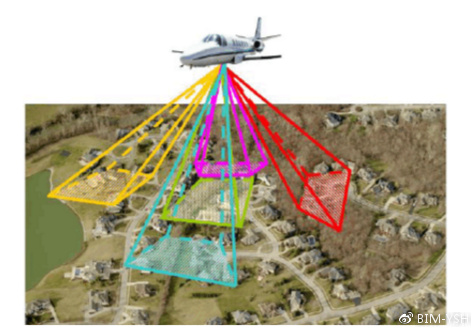使用大疆无人机进行数据，可以用单镜头模拟多镜头成像，规划5条航线，分五次飞行采集数据。

​在建立建筑物表面模型的过程中，下图可以看到，相比垂直影像，倾斜影像有着显著的优点，因为它能提供更好的视角去观察建筑物侧面，这一特点正好满足了建筑物表面纹理生成的需要。同一区域拍摄的垂直影像可被用来生成三维城市模型或是对生成的三维城市模型的改善。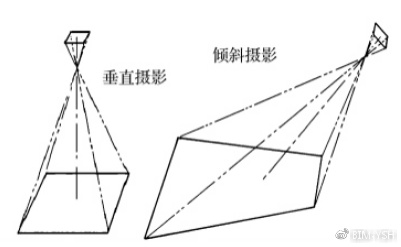刚接触的Pix4Dcapture飞控软件，跟飞友们分享一下，相互学习：

飞机基础选项
当我们打开软件时，会进入到开始界面，左上角是飞机型号选项和其他参数设置，右上交是推出选项。点击左上角，进行选择我们所用的飞机型号，与一些基础设置参数调选。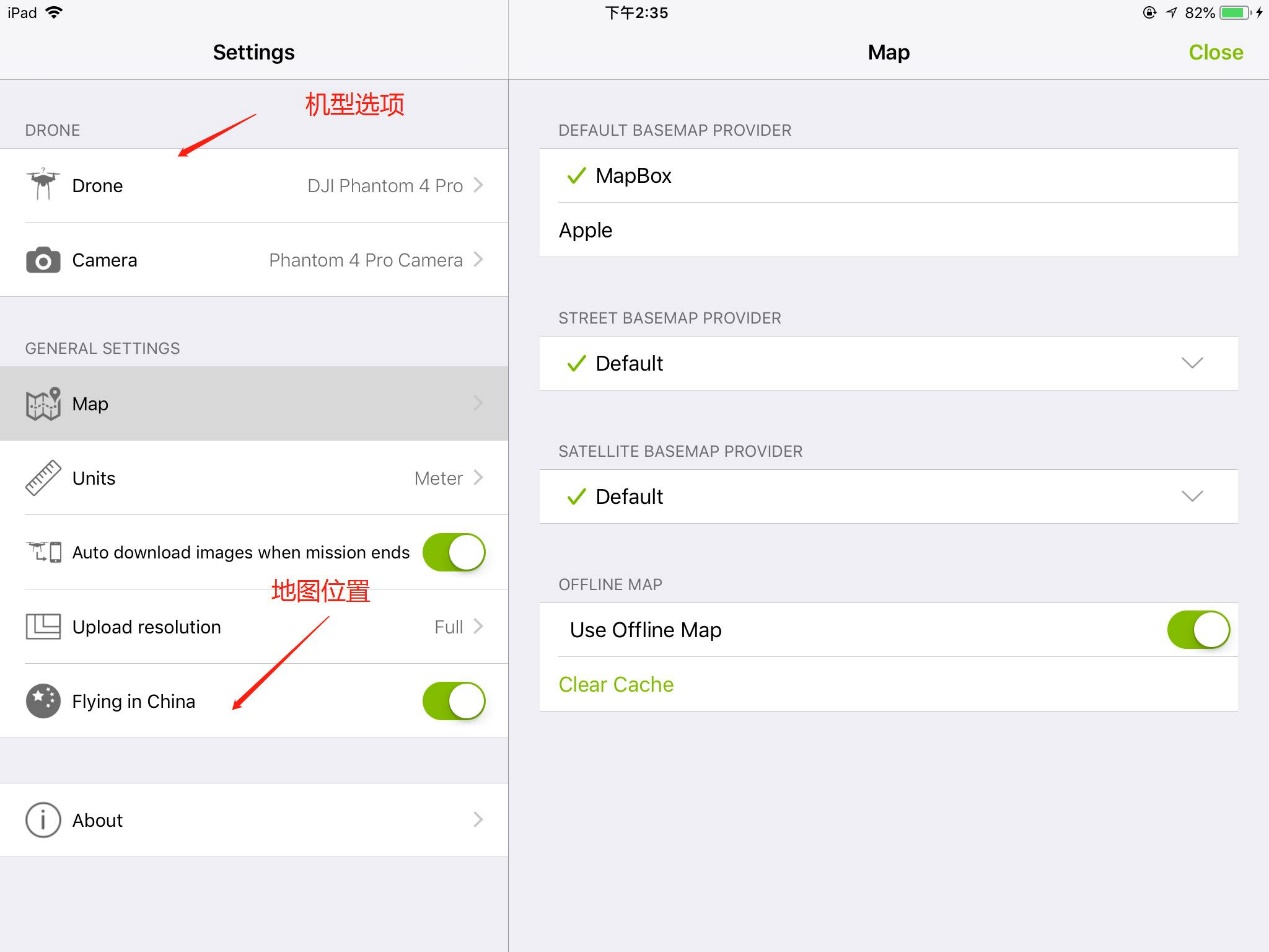2、飞行选项

中间是飞行模式有五种，从左往右，第一个是多边形2D地图，第二个是网格2D地图，第三个是双网格3D模型，第四个是循环对于单3D模型，第五个是免费航班高级用户。左下角是项目列表选项，右下角是教程帮助选项。一般我们常用的是双网格3D模型和循环对于单3D模型。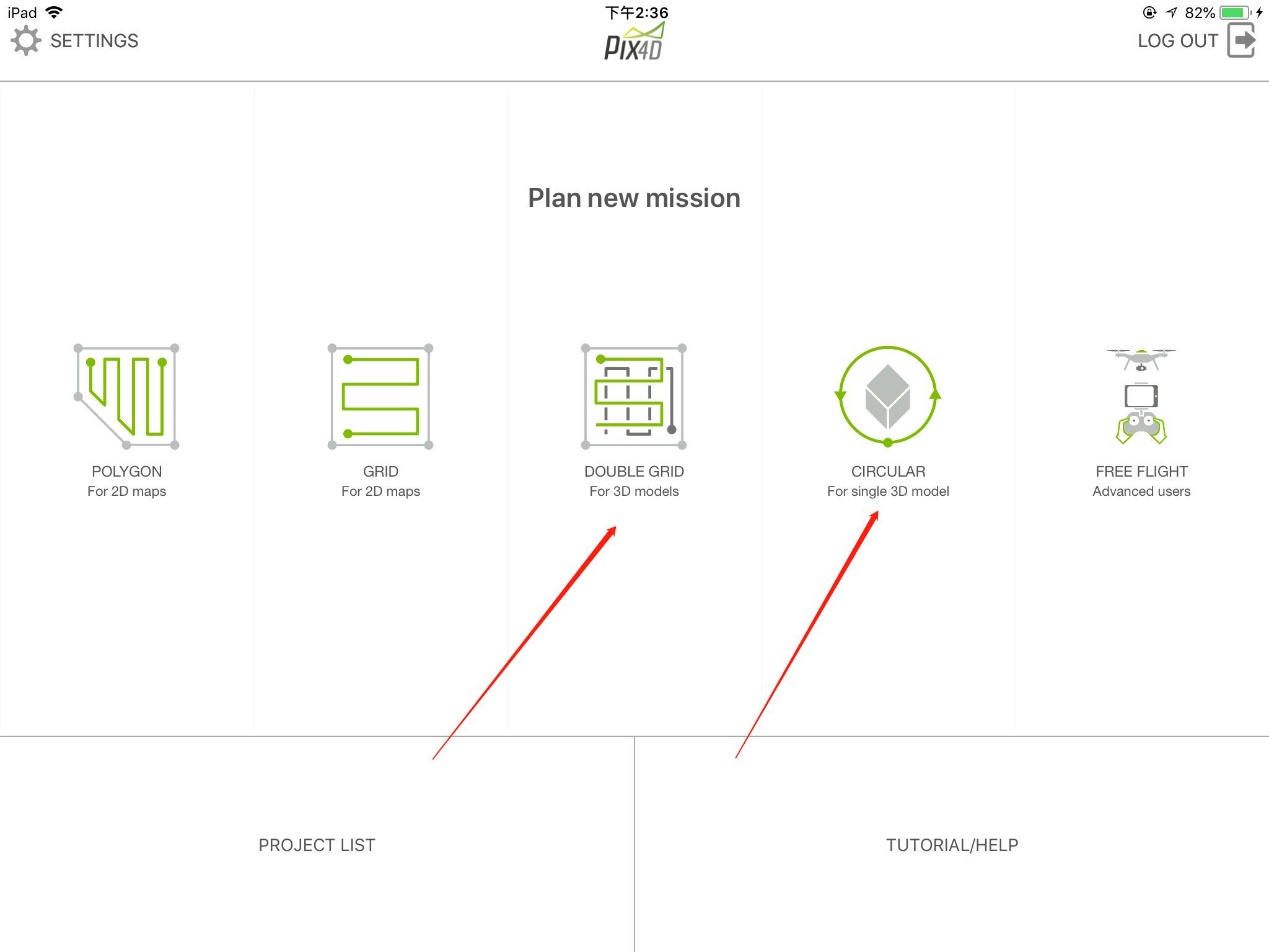规划航线
一般我们选相机角度是45度，所以在画航线时，要超出所测的范围，这样才能把所测局域拍全。飞行高度要超过飞行区域内所有障碍物，以免飞行过程中撞高建筑物，造成飞机炸毁的后果，飞友特别注意。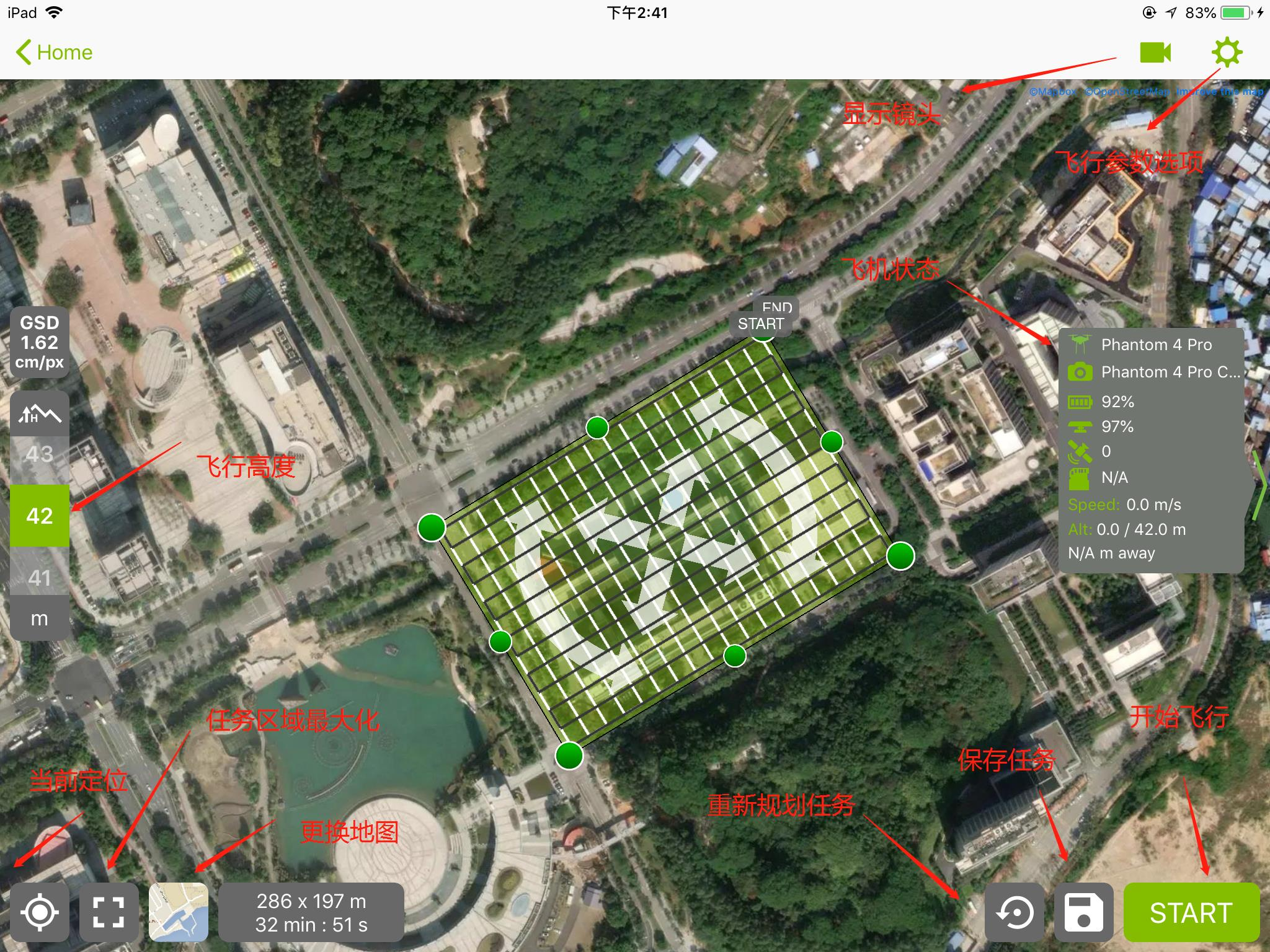飞行设置
一般相机角度我们选择是45度角
前重叠度和侧面重叠度设置70%（最好不要低于70%，高精度建模可以调到90%，飞行的时间会增加）
飞行速度一般选最大，它也不会飞到我们所测的飞速度。
查看中心看个人喜好是否要打开。
相机触发模式有安全模式和快速模式，一般我选快速模式（安全模式下，飞行器拍摄照片时会悬停，当图传丢失时，会继续执行拍照任务；快速模式下，飞行器边飞边拍摄照片，但是当图传丢失时，拍照任务会停止）。
白平衡可根据当地天气来调选。
忽略homepoint可以默认选择关闭（虽然软件提供忽略起飞点安全检查项设置，但是经实际测试，飞行器位置离测区中心点超过150米，还是无法起飞)。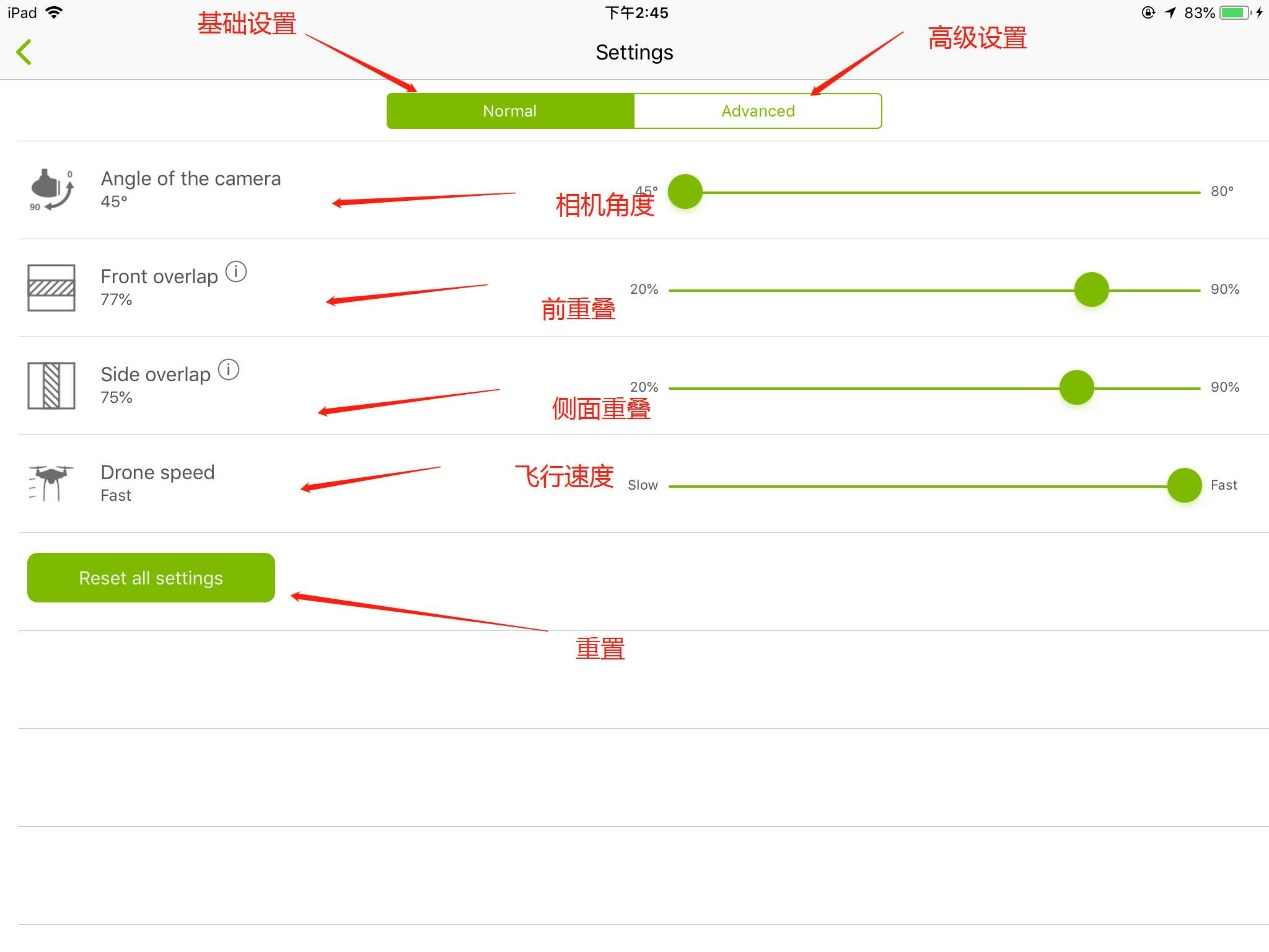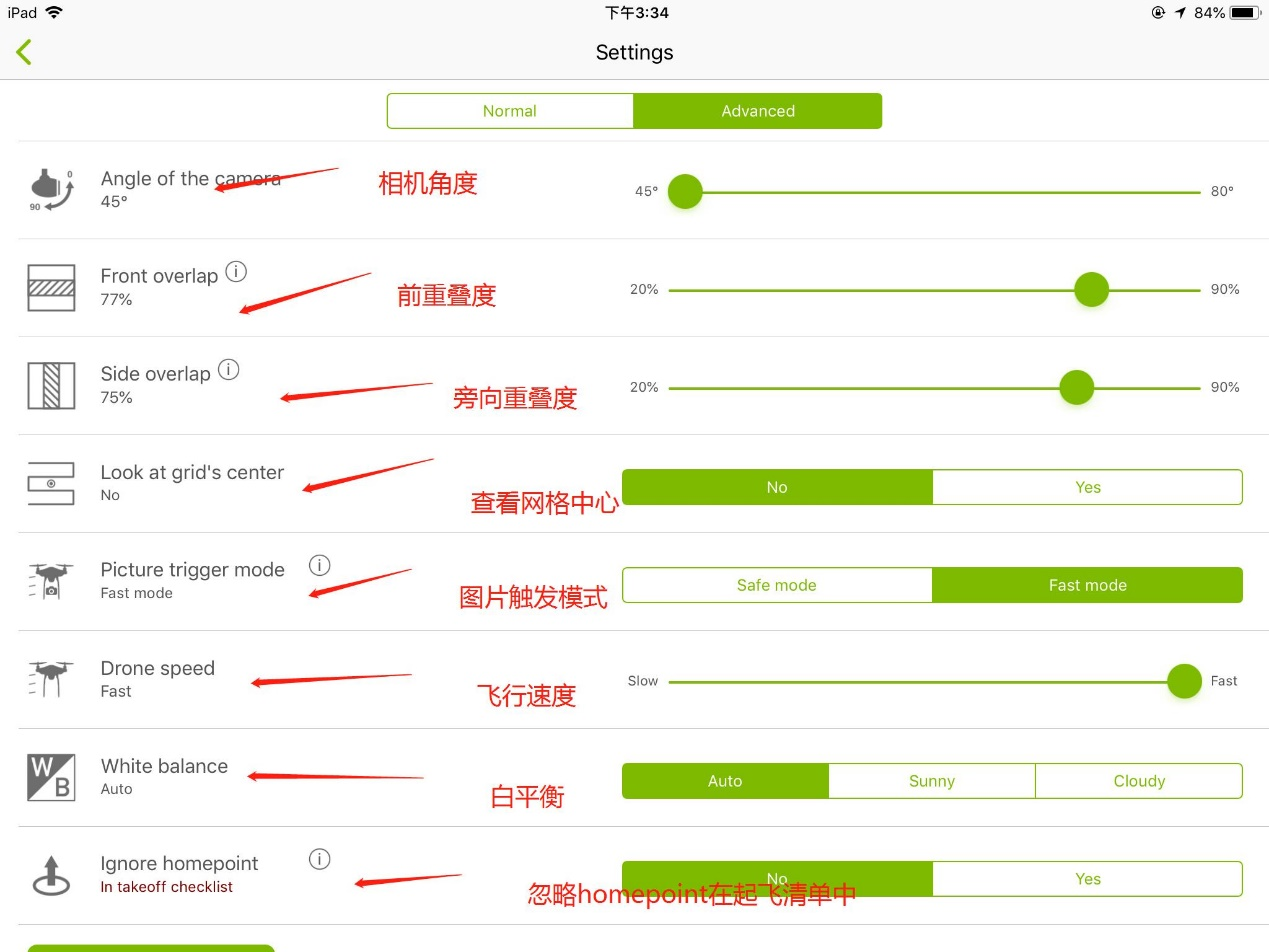当任务设置好后点击保存，开始执行任务
当我们点击开始执行任务是，飞机会弹出以下对话框，飞机进行自检：无人机连接情况、电池电量、GPS定位情况、摄像机状态、返航点位置、无人机是否靠近测区、遥控器档位设置等。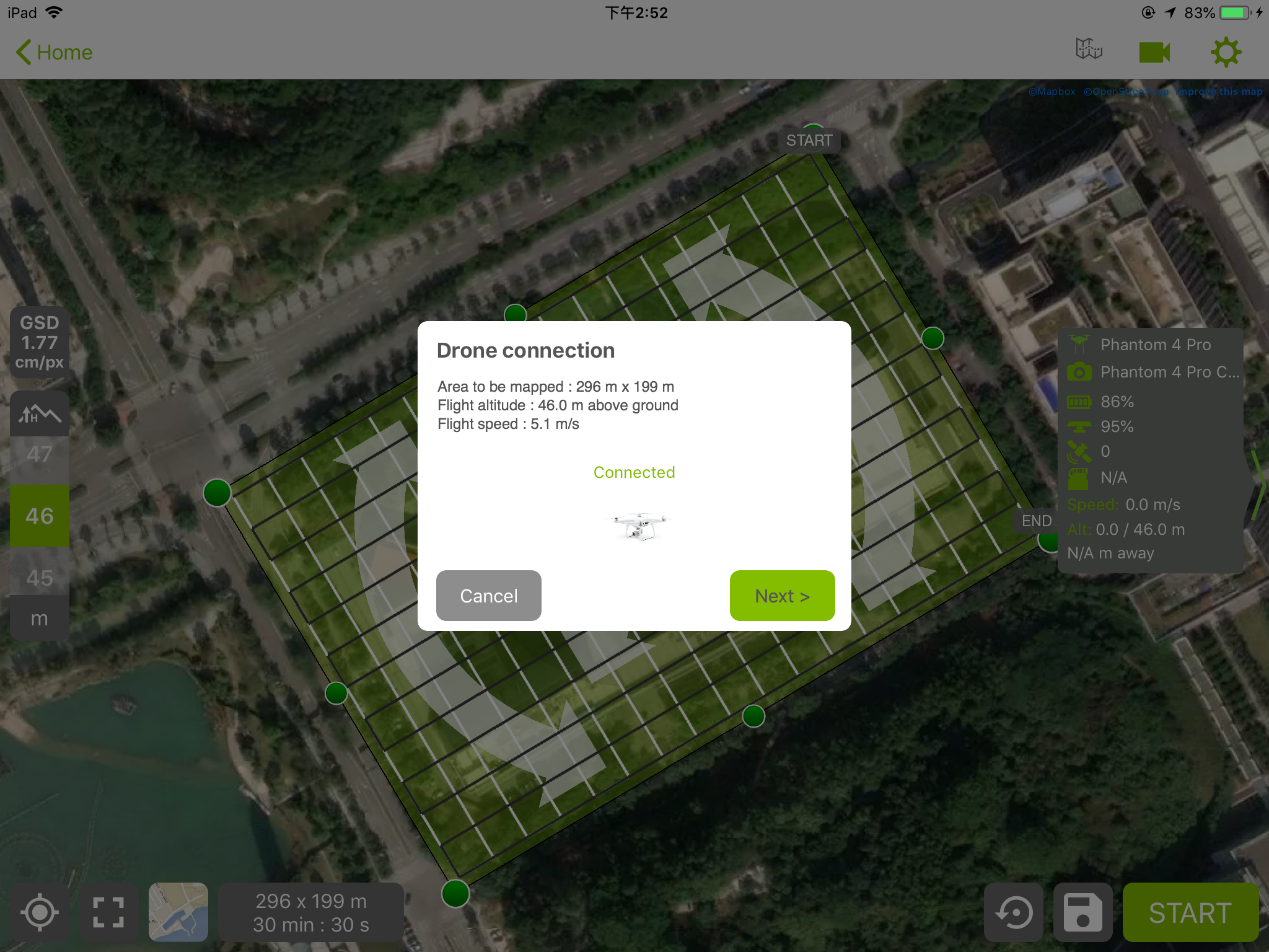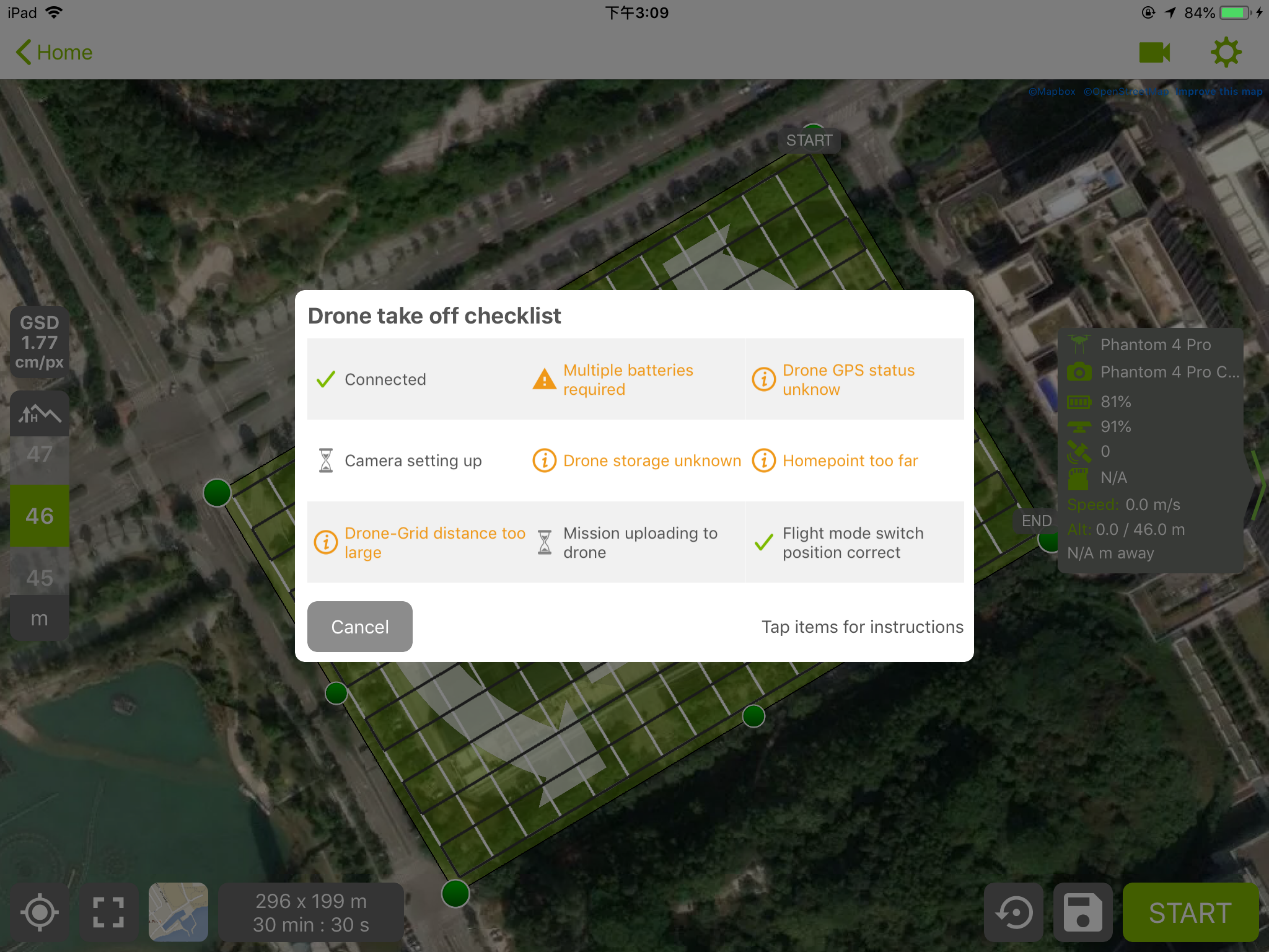6、当飞机检查通过后，就可以点击开始飞行了。

注意事项：

（1）预计飞行时间功能：支持。

（2） 低电量报警功能：支持。

（3）低电量报警功能：支持。（低电量（30%）自动报警功能，和DJI GO4功能一样）

（4）自动返航功能：支持。（飞行过程中，APP会根据无人机与返航点的距离来计算返航电量，达到需要返航的电量时，会立即自动返航。）

（5） 断点续航功能：不支持。（特别注意任务所需要的时间，与飞机飞行的时间，看能不能控制飞行器在一个架次内完成两个互相垂直的格网航线，要不然徒劳无功）

（6） 失控返航功能：支持。

（7）其它安全策略：起飞点需处于测区中心位置（最远距离不超过150米），否则起飞前安全检查时会判定Home点过远无法起飞。

利用建模软件将照片建模，这里的照片不仅仅是通过无人机航拍的倾斜摄影数据，还可以是单反甚至是手机以一定重叠度环拍而来的，这些照片导入到建模软件中，通过计算机图形计算，结合pos信息空三处理，生成点云，点云构成格网，格网结合照片生成赋有纹理的三维模型。区域整体三维建模方法生产路线图：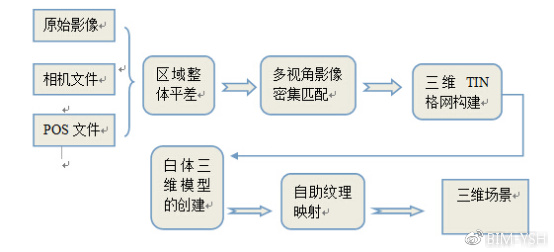行业里主流的有Smart3D、Photoscan、 Pix4D mapper等这几个建模软件也是各有优缺点，Photoscan比较轻量级，但是生成的模型纹理效果不是太理想，Smart3D生成的三维模型效果最为理想，人工修复工作量较低，但是软件比较复杂不易上手且价格较高。目前国内企业科创数字的“如初三维重建平台”，可以不需要有专业背景，测量设备、不需要手动贴图，让场景重建变得更简单，你只需要拍摄一些照片即可，上传到平台即可。

如初三维重建平台的研发而起无疑造福了大量的三维爱好者，使得三维重建的门槛大大降低，人人都可以成为三维模型的作者。下面我们来看怎么在“如初三维重建平台”制作模型。

总结

在如初三维建模平台上面注册免费账号上传照片，等待云端自动生成3D模型。Smart3D、Photoscan建模对电脑要求很高，费时费力，还不一定能成，直接免费云端建模效率超高，还不用浪费电费，推荐大家使用。其实我刚才看了个图文并茂的3D建模帖子，觉得其实没有必要，直接使用云端才好。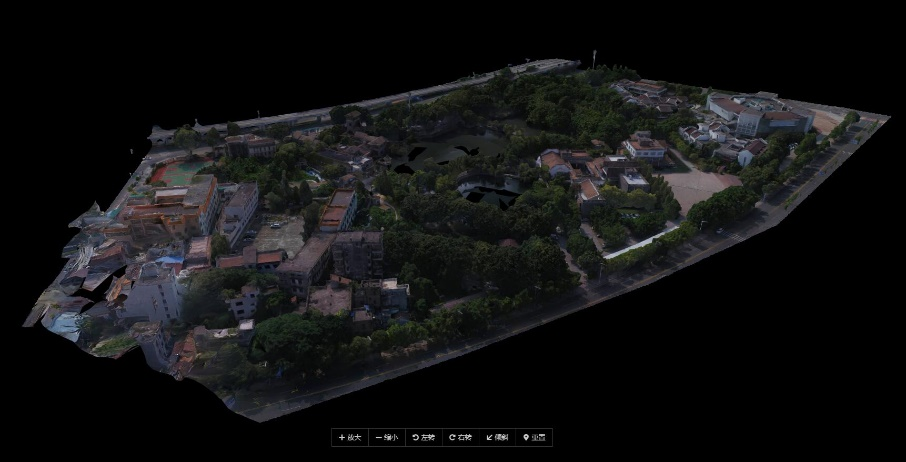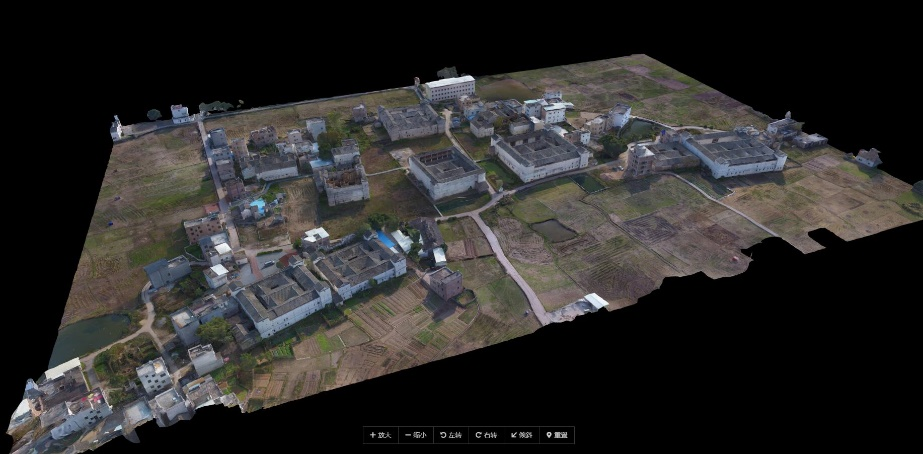展开全文数字化建模
• 上篇博客简要的介绍了多旋翼飞行器的建模过程，但是在动力学建模过程中，我们忽略了比较重要的陀螺力矩；电机高速旋转的时候，相当于一个陀螺，高速旋转的陀螺是非常稳定的个体，具有保持自身轴向不变的能力(定轴性)...
上篇博客简要的介绍了多旋翼飞行器的建模过程，但是在动力学建模过程中，我们忽略了比较重要的陀螺力矩；电机高速旋转的时候，相当于一个陀螺，高速旋转的陀螺是非常稳定的个体，具有保持自身轴向不变的能力(定轴性)，因此如果有外力想改变陀螺转轴的方向，那么会产生一个陀螺力矩来抵抗这种改变。
下面来看考虑陀螺力矩的动力学方程：
$\mathbf{J} \cdot^{b} \dot{\boldsymbol{\omega}}=-^{b} \boldsymbol{\omega} \times\left(\mathbf{J} \cdot^{b} \boldsymbol \omega\right)+\mathbf{G}_{a}+\boldsymbol{\tau}$
$\mathbf{G}_{a}$表示陀螺力矩
$\mathbf{G}_{a}=\boldsymbol{J}_{R P}\mathbf \Omega \times \boldsymbol{\omega}$
其中$\mathbf \Omega$为螺旋桨角速向量。$\boldsymbol{\omega}$表示电机转轴的旋转角速度，我们认为转轴角速度等于机体角速度。
旋翼组件k(含电机和螺旋桨)的陀螺力矩为：
$\mathbf{G}_{a,k}=J_{R P}\mathbf \Omega_{k} \mathbf b_{3} \times \boldsymbol \omega$
其中$\mathbf b_3$为螺旋桨角速度方向，因为在机体坐标系中，螺旋桨只有$z$轴方向有角速度所以， $\mathbf b_3$为$[0,0,1]^T$ , $J_{RP}$ 为电机转子和螺旋桨绕轴的总转动惯量。
其中
$\mathbf b_{3} \times \boldsymbol \omega=\mathbf b_{3}^{\times} \boldsymbol \omega=-\boldsymbol \omega^{\times}\mathbf b_{3}$
$\times$表示叉乘算子。即：
$\boldsymbol \omega=\left[ \begin{array}{c}{\omega_{x}} \\ {w_{y}} \\ {w_{z}}\end{array}\right] \Longleftrightarrow \left[ \begin{array}{ccc}{0} & {-w_{z}} & {w_{y}} \\ {w_{z}} & {0} & {-w_{x}} \\ {-w_{y}} & {w_{x}} & {0}\end{array}\right]=\boldsymbol \omega^{\times}$
则有：
$\mathbf{G}_{a,k}=-J_{R P} \mathbf\Omega_{k} \left[ \begin{array}{c}{w_{y}} \\ {-w_{x}} \\ {0}\end{array}\right]$
其中$\mathbf \Omega _k$为第k个螺旋桨角速度，根据右手定则，机体z轴的旋转正方向为顺时针。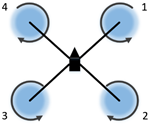$k=1$时，螺旋桨转速为负，$\mathbf \Omega _1 = -\Omega _1$，其余螺旋桨的转速以此类推；最终得到陀螺力矩$\mathbf{G}_{a}$的表达形式如下：
$\left\{\begin{array}{l}{{G}_{ax}=J_{R P} \omega_{y}\left(\Omega_{1}-\Omega_{2}+\Omega_{3}-\Omega_{4}\right)} \\ {{G}_{ay}=J_{R P} \omega_{x}\left(-\Omega_{1}+\Omega_{2}-\Omega_{3}+\Omega_{4}\right)} \\ {{G}_{az}=0}\end{array}\right.$


展开全文• 在过去的几年中，无人机系统被广泛应用于各种民用领域，其中一些应用需要精确的制导和控制。因此，无人机的制导控制吸引了控制理论和航天工程领域的众多研究者。飞翼作为一种特殊的无人机，被认为是最有效的气动结构...
• 无人机四旋翼建模 近期需要学习考虑四旋翼建模的知识，但是苦于找不到现成的matlab模块来学习，经过长时间的寻找终于在matlab的论坛上找到了一些资源现在把他共享出来 –此文件并不是我本人所写，下面提供连接 链接 ...经验分享
• 使用python推导雅可比矩阵 使用python进行建模无人机PX4中EKF模型的生成
• 一篇写得很好很全面的无人机建模和计算机（XML）仿真实现的学位论文
• 参考1：如何用大疆无人机三维建模之最简单办法一、拍摄使用DJI GS PRO，altizure，pix4dmapper capture 等采集数据，这三个app都很好用，采集数据都是自动完成的，不必细说。二、在altizure或者dronedeploy上面注册...
• 来源 引自：Modeling, Simulation and Control System Design for Civil Unmanned Aerial Vehicle (UAV) 2. 建模github
• 项目需要调研一下无人机三维建模的信息无人机三维建模核心技术是三维重建，或者说基于图片的建模（Image-Based Modeling）。项目需要是建立园区的三维模型，其他应用上可以用于古街道、文物建模。（参考...
•研究论文
• 由于在线建模具有建模完成时间不确定，采用本机建模的方式，这里使用Photoscan建模。我用的是1.4.0破解版，破解很简单，下载包里就有一个exe，覆盖安装目录中的文件就行。Agisoft PhotoScan软件介绍 Agisoft Photo...
• 我从2017年8月份开始接触无人机的，到现在玩无人机已经差不多有一年了。玩无人机的初衷是为了出去旅游时，可以记录一下一些美好的瞬间以及完美的场景。以前去旅游时，只带相机去拍照，局限性就很大，好多很好的场景...数字化建模 数字模型
• 转载于:https://www.cnblogs.com/lolitagis02/p/8148730.html
•研究论文
• 在军用和民用方面具有较大的潜在应用价值，国内外许多研究单位纷纷致力于四旋翼无人机飞行控制的架构设计与飞行控制研究，以实现四旋翼无人机的自主飞行。机载传感器系统是四旋翼无人机飞行控制系统的重要组成部分，...数字化建模
• 【倾斜摄影三维建模技术简介】倾斜摄影技术是国际测绘领域近年来发展起来的一项高新技术，颠覆了以往正射影像只能从垂直角度拍摄的局限，通过在同一飞行平台上搭载多台传感器，同时从1个垂直、4个倾斜共5个不同的...
• 即可全自动一键操作，不需要人为交互处理无人机数据。 自动生成点云、纹理、网格，无需人为去干涉，不用后期贴图处理。飞机操控员就能够直接处理和查看结果，并把结果发送给最终用户。 不占计算机资源 “如...三维建模 数字化建模
• 新型同轴八旋翼无人机的自适应RBFNN建模和鲁棒反推滑模控制
• ContextCapture，原名Smart3D FR:徐海涛（hunk Xu) QQ技术交流群：386476712
• 随着无人机倾斜摄影测绘技术的不断深入和发展，为城市实景三维建模提供了新的技术路径，在智慧城市实景三维建模中具有重要的应用优势和价值。通过实例分析，结合多种建模软件，探讨了无人机倾斜摄影测绘技术在智慧...
• 摘 要针对于虚拟现实平台中构建三维场景的费时费力问题，基于无人机倾斜摄影建模技术构建三维模型，利用 3DS Max 建模软件进行模型优化，并结合 Unity 3D 引擎构建并完成了虚拟校园平台的开发。实践结果表明，构建的...
• 基于无人机影像的三维建模及正射影像精度评估，刘宇，郑新奇，近年来，许多国家在摄影测量基数领域中对无人机技术的兴趣浓厚。无人机灵活度高、机动性好、周期短，是快速获取高分辨率影像的重
•研究论文
•研究论文...